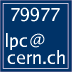Luminosity and PU conditions during the intensity ramp up in 2022 LPC homeThe parameters on this page reflect the current best guess of the machine conditions and will be regularly updated. Please consider this when using these parameters for any estimate. In case of doubts do not hesitate to ask the LPC.

## Intensity Ramp Up 2022

We project the instantaneous luminosity (in units of cm-2s-1) and pile up to be expected during the intensity ramp up of the LHC in the following tables, with varying assumptions on β* and beam emittance (based on the LHC beam parameters archieved in early July 2022).

The numbers below assume a bunch intensity of 1.2 x 1011 ppb (protons per bunch).

The situation at the beginning of the fill (β* = 60 cm) would be the following:

β* = 60 cm
Emittance \ #bunches 3b 12b 75b 300b 600b 900b 1200b 1500b
ε=1.8 μm L =1.1 x 1031
PU = 40
L =4.5 x 1031
PU = 40
L =3.5 x 1032
PU = 40
L =1.8 x 1033
PU = 40
L =3.3 x 1033
PU = 40
L =5.5 x 1033
PU = 40
L =6.8 x 1033
PU = 40
L =8.6 x 1033
PU = 40
ε=2.1 μm L =1031
PU = 36
L =4 x 1031
PU = 36
L =3.1 x 1032
PU = 36
L =1.6 x 1033
PU = 36
L =3 x 1033
PU = 36
L =4.9 x 1033
PU = 36
L =6 x 1033
PU = 36
L =7.7 x 1033
PU = 36
ε=2.3 μm L =0.9 x 1031
PU = 33
L =3.7 x 1031
PU = 33
L =2.9 x 1032
PU = 33
L =1.5 x 1033
PU = 33
L =2.7 x 1033
PU = 33
L =4.6 x 1033
PU = 33
L =5.7 x 1033
PU = 33
L =7.2 x 1033
PU = 33

Assuming fast leveling to β* = 45 cm, one would get the following:

β* = 45 cm
Emittance \ #bunches 3b 12b 75b 300b 600b 900b 1200b 1500b
ε=1.8 μm L =1.4 x 1031
PU = 49
L =5.4 x 1031
PU = 49
L =4.2 x 1032
PU = 49
L =2.2 x 1033
PU = 49
L =4 x 1033
PU = 49
L =6.7 x 1033
PU = 49
L =8.3 x 1033
PU = 49
L =1 x 1034
PU = 49
ε=2.1 μm L =1.3 x 1031
PU = 44
L =4.9 x 1031
PU = 44
L =3.8 x 1032
PU = 44
L =2 x 1033
PU = 44
L =3.6 x 1033
PU = 44
L =6 x 1033
PU = 44
L =7.5 x 1033
PU = 44
L =9.5 x 1033
PU = 44
ε=2.3 μm L =1.1 x 1031
PU = 41
L =4.6 x 1031
PU = 41
L =3.6 x 1032
PU = 41
L =1.9 x 1033
PU = 41
L =3.4 x 1033
PU = 41
L =5.6 x 1033
PU = 41
L =7 x 1033
PU = 41
L =8.9 x 1033
PU = 41

Assuming fast leveling to β* = 30 cm, one would get the following:

β* = 30 cm
Emittance \ #bunches 3b 12b 75b 300b 600b 900b 1200b 1500b
ε=1.8 μm L =1.8 x 1031
PU = 63
L =7.1 x 1031
PU = 63
L =5.5 x 1032
PU = 63
L =2.9 x 1033
PU = 63
L =5.3 x 1033
PU = 63
L =8.7 x 1033
PU = 63
L =1.1 x 1034
PU = 63
L =1.4 x 1034
PU = 63
ε=2.1 μm L =1.6 x 1031
PU = 57
L =6.5 x 1031
PU = 57
L =5 x 1032
PU = 57
L =2.6 x 1033
PU = 57
L =4.8 x 1033
PU = 57
L =7.9 x 1033
PU = 57
L =9.8 x 1033
PU = 57
L =1.3 x 1034
PU = 57
ε=2.3 μm L =1.5 x 1031
PU = 54
L =6.1 x 1031
PU = 54
L =4.7 x 1032
PU = 54
L =2.5 x 1033
PU = 54
L =4.5 x 1033
PU = 54
L =7.4 x 1033
PU = 54
L =9.2 x 1033
PU = 54
L =1.2 x 1034
PU = 54

For a bunch intensity of 1.4 x 1011 ppb, the above numbers should be multiplied by a factor 1.36.

This web site is owned by the account lpc .